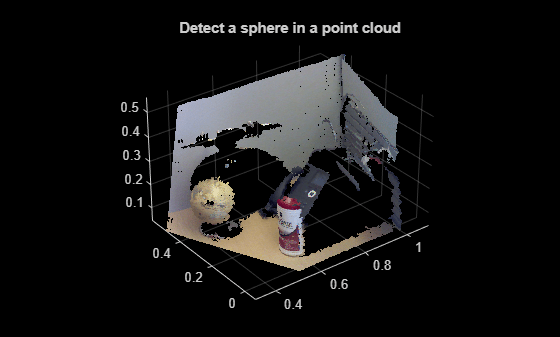# plot

Plot parametric model

## Syntax

``plot(model)``
``plot(model,Name=Value)``
``H = plot(___)``

## Description

example

````plot(model)` plots a model within the axis limits of the current figure. `H` is the handle to `surf`, a 3-D shaded surface plot or `patch`, a filled polygon region.```
````plot(model,Name=Value)` specifies options using one or more name-value arguments in addition to argument from previous syntax. For example, `plot(model,Color="r")` sets the color of the plot to red.```
````H = plot(___)` specifies options using one or more name-value arguments. For example, `plot(model,Color="r")` sets the color of the plot to red.```

## Examples

collapse all

Load a MAT file containing a point cloud into the workspace.

`load("object3d.mat");`

Display the point cloud.

```figure pcshow(ptCloud) xlabel("X(m)") ylabel("Y(m)") zlabel("Z(m)") title("Detect a Cylinder in a Point Cloud")```

Set the maximum point-to-cylinder distance for cylinder fitting to 5mm.

`maxDistance = 0.005;`

Specify a region of interest (ROI) to constrain the fitting function.

```roi = [0.4 0.6; -inf 0.2; 0.1 inf]; sampleIndices = findPointsInROI(ptCloud,roi);```

Set the orientation constraint for the fitting function

`referenceVector = [0 0 1];`

Detect the cylinder in the specified ROI of the point cloud and extract it.

```model = pcfitcylinder(ptCloud,maxDistance,referenceVector, ... SampleIndices=sampleIndices);```

Plot the model of the detected cylinder.

```hold on plot(model)```Load a MAT file containing a point cloud.

`load("object3d.mat");`

Display the point cloud into the workspace.

```figure pcshow(ptCloud) xlabel("X(m)") ylabel("Y(m)") zlabel("Z(m)") title("Detect a Plane in a Point Cloud")```

Set the maximum point-to-plane distance for plane fitting to 2cm.

`maxDistance = 0.02;`

Specify the normal vector plane for the fitted plane.

`referenceVector = [0 0 1];`

Set the maximum angular distance for the fitted plane to 5 degrees.

`maxAngularDistance = 5;`

Detect the plane, the table, in the point cloud and extract it.

`model = pcfitplane(ptCloud,maxDistance,referenceVector,maxAngularDistance);`

Plot the plane.

```hold on plot(model)````load("object3d.mat");`

Display point cloud.

```figure pcshow(ptCloud) xlabel("X(m)") ylabel("Y(m)") zlabel("Z(m)") title("Detect a sphere in a point cloud")```Set the maximum point-to-sphere distance (1cm), for sphere fitting.

`maxDistance = 0.01;`

Set the region of interest to constrain the search.

```roi = [-inf,0.5;0.2,0.4;0.1,inf]; sampleIndices = findPointsInROI(ptCloud,roi);```

Detect the globe in the point cloud and extract it.

`model = pcfitsphere(ptCloud,maxDistance,SampleIndices=sampleIndices);`

Plot the sphere.

```hold on plot(model)```## Input Arguments

collapse all

Parametric model returned by the `cylinderModel`, `planeModel`, or the `sphereModel` object.

### Name-Value Arguments

Specify optional pairs of arguments as `Name1=Value1,...,NameN=ValueN`, where `Name` is the argument name and `Value` is the corresponding value. Name-value arguments must appear after other arguments, but the order of the pairs does not matter.

Example: `plot(model,Color="r")` sets the color of the plot to red.

Output axes, specified as the current axes for displaying the visualization.

Color of the plane, specified as a 1-by-3 RGB vector or a short or long color name.

Color NameShort NameRGB TripletAppearance
`'red'``'r'``[1 0 0]``'green'``'g'``[0 1 0]``'blue'``'b'``[0 0 1]``'cyan'` `'c'``[0 1 1]``'magenta'``'m'``[1 0 1]``'yellow'``'y'``[1 1 0]``'black'``'k'``[0 0 0]``'white'``'w'``[1 1 1]`## Output Arguments

collapse all

Handle to a plot, returned as a `surf` 3-D shaded surface plot or as a `patch` filled polygon region.

## Version History

Introduced in R2015b